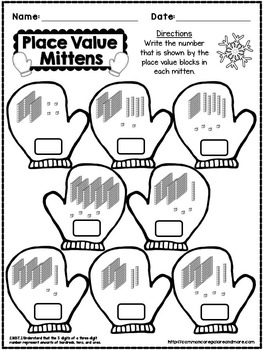Winter No Prep Math - 2nd Grade1st - 3rd, Homeschool
Subjects
Standards
Resource Type
Formats Included
• PDF
Pages
5 pages

Description

Winter No Prep Math - 2nd Grade

This is a FREE 5 PAGE SAMPLE of the following product:
Winter Math Pack for 2nd Grade

This SAMPLE PACK includes:
Let’s Skate! (1 page) - Solve each addition equation, then use the color code to color the skates. (2.OA.2 and 2.OA.3)
Winter Facts (1 page) - Solve each subtraction equation, then use the color code to color the tags. (2.OA.2 and 2.OA.3)
Winter Arrays (1 page) - Write the number of rows and columns under each winter array. (2.OA.4)
Place Value Mittens (1 page) - Write the number that is shown by the place value blocks in each mitten. (2.NBT.1)
Winter Shapes! (1 page) - Use the code to color the shapes in each snow globe. (2.G.1)

This 50 page Winter Math Pack requires NO PREP. All you need to do is hit print and go! Every page is aligned to the Common Core Math Standards for Grade 2. I included the standard covered at the bottom of each page.

This pack includes the following printables:
Word Problems (2 pages) - Solve winter themed word problems. (2.OA.1)
Mystery Number (2 pages) - Solve the addition equations, then color the boxes to find the mystery number. (2.OA.2)
Let’s Skate! (2 pages) - Solve each addition equation, then use the color code to color the skates. (2.OA.2 and 2.OA.3)
Winter Facts (2 pages) - Solve each subtraction equation, then use the color code to color the tags. (2.OA.2 and 2.OA.3)
Winter Arrays (2 pages) - Write the number of rows and columns under each winter array. (2.OA.4)
Winter Totals (2 pages) - Find the total of each array by writing 2 equations: one showing the total as a sum by rows and one showing the total as a sum by columns. (2.OA.4)
Place Value Mittens (4 pages) - Write the number that is shown by the place value blocks in each mitten. (2.NBT.1)
Winter Numbers (4 pages) - Write each number in written form. (2.NBT.3)
Build and Draw! (4 pages) - Build each number using base 10 blocks, then add up the tens and ones to find the total. (2.NBT.5)
Add and Color! (1 page) - Solve each addition equation, then use the color code to color the mittens. (2.NBT.5)
Let’s Subtract! (1 page) - Solve each subtraction equation, then use the color code to color the mittens. (2.NBT.5)
More and Less (4 pages) - Look at the number in each box, then use the color code to color each pie. (2.NBT.8)
Snowy Tens (4 pages) - Write the number that is 10 more and 10 less than the number in each snowball. (2.NBT.8)
Snowy Hundreds (4 pages) - Write the number that is 100 more and 100 less than the number in each snowball. (2.NBT.8)
Cocoa Clocks! (4 pages) - Color the matching analog and digital clocks the same color. (2.MD.7)
Getting Digital! (4 pages) - Write the digital time under each analog clock. (2.MD.7)
Money Word Problems (2 pages) - Solve winter themed money word problems. (2.MD.8)
Winter Shapes! (2 pages) - Use the code to color the shapes in each snow globe. (2.G.1)

•••••••••••••••••••••••••••••••••••••••••••••••••••••••••••••••••••••••••••••••••••

If you are interested in purchasing every Common Core Math resource in my store for 2nd grade at a discounted price, please use the following link:
Common Core Math Resources for Grade 2

•••••••••••••••••••••••••••••••••••••••••••••••••••••••••••••••••••••••••••••••••••

Here are some other 2nd grade products that you may be interested in:
2nd Grade Common Core Math Assessments for ALL Standards
Fall Prepless Printables for 2nd Grade
October NO PREP Math Pack
2nd Grade Common Core Math Story Problems Bundle
Tricky Word Problems for 2nd Grade
Fall Fun Math Pack
Common Core Array Activities Bundle
Common Core Shape Activities
Place Value Match Game
Ways to Make Money
2-Step Word Problems

•••••••••••••••••••••••••••••••••••••••••••••••••••••••••••••••••••••••••••••••••••

Be the first to know about my new discounts, freebies and product launches:
♦ Look for the green star near the top of any page within my store and click it to become a follower. You will then receive customized email updates about my store.

•••••••••••••••••••••••••••••••••••••••••••••••••••••••••••••••••••••••••••••••••••

Thank you for stopping by! :)
Pinterest
Common Core Galore and More's Blog
Total Pages
5 pages
Not Included
Teaching Duration
N/A
Report this Resource to TpT
Reported resources will be reviewed by our team. Report this resource to let us know if this resource violates TpT’s content guidelines.

Standards

to see state-specific standards (only available in the US).
Recognize and draw shapes having specified attributes, such as a given number of angles or a given number of equal faces. Identify triangles, quadrilaterals, pentagons, hexagons, and cubes.
Use addition to find the total number of objects arranged in rectangular arrays with up to 5 rows and up to 5 columns; write an equation to express the total as a sum of equal addends.
Determine whether a group of objects (up to 20) has an odd or even number of members, e.g., by pairing objects or counting them by 2s; write an equation to express an even number as a sum of two equal addends.
Fluently add and subtract within 20 using mental strategies. By end of Grade 2, know from memory all sums of two one-digit numbers.
The numbers 100, 200, 300, 400, 500, 600, 700, 800, 900 refer to one, two, three, four, five, six, seven, eight, or nine hundreds (and 0 tens and 0 ones).